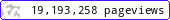•••••MadAsMaths.com :: Maths Booklets :: Further Topics :: Various

FURTHER TOPICS - VARIOUS

These booklets are suitable for

• students taking A Level Further Mathematics.

• introduction/revision/practice to more advanced mathematical topics, except integration and linear algebra, for undergraduates in science degrees, science diplomas or mathematics degrees.

Please click on the titles below to download the files

 advanced_mensuration.pdfcombinatorics.pdfcomplex_numbers_part_1.pdfcomplex_numbers_part_1_exam_questions.pdfcomplex_numbers_part_2.pdfcomplex_numbers_part_2_exam_questions.pdfconic_sections.pdfcurve_sketching.pdfdifferentiation_from_first_principles.pdfdifferentiation_practice_iii.pdfgroups_and_relations.pdfhyperbolic_functions.pdflinearization_of_graphs.pdfnumber_theory.pdfpolar_coordinates_exam_questions.pdfpolar_coordinates_practice.pdfproof_by_induction.pdfproof_general.pdfrational_functions.pdfroots_of_polynomial_equations.pdfseries_and_integrals.pdfseries_expansions.pdfseries_summation_practice.pdf